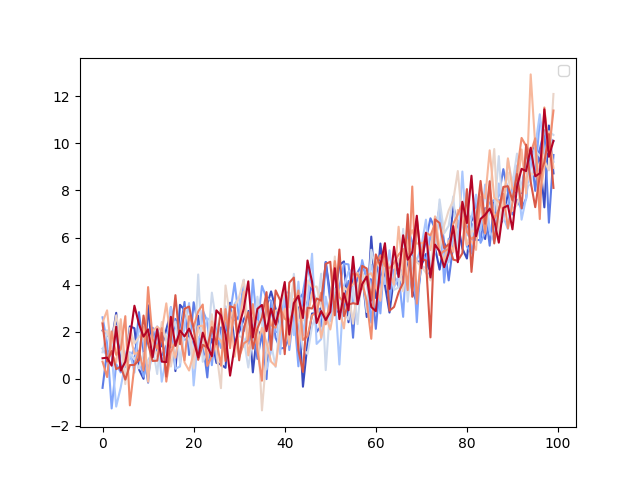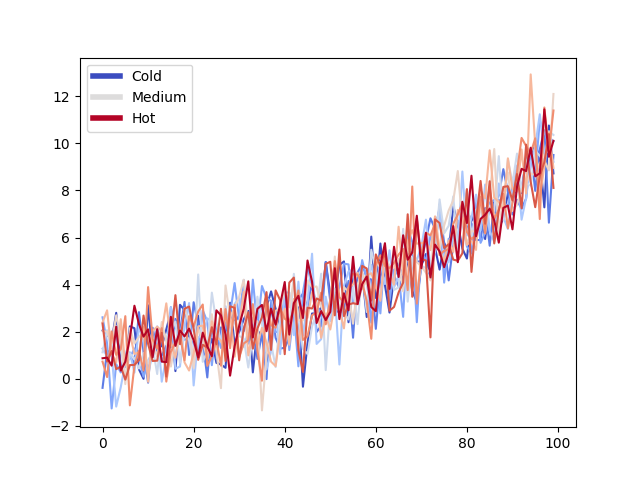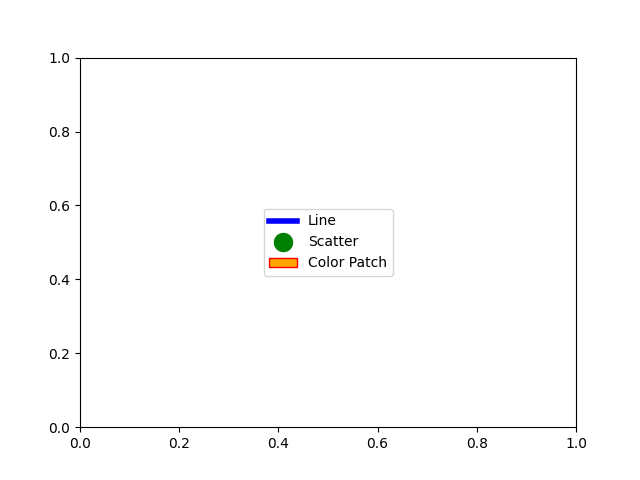# Composing Custom Legends¶

Composing custom legends piece-by-piece.

Note

For more information on creating and customizing legends, see the following pages:

Sometimes you don't want a legend that is explicitly tied to data that you have plotted. For example, say you have plotted 10 lines, but don't want a legend item to show up for each one. If you simply plot the lines and call ax.legend(), you will get the following:

from matplotlib import rcParams, cycler
import matplotlib.pyplot as plt
import numpy as np

# Fixing random state for reproducibility
np.random.seed(19680801)

N = 10
data = (np.geomspace(1, 10, 100) + np.random.randn(N, 100)).T
cmap = plt.cm.coolwarm
rcParams['axes.prop_cycle'] = cycler(color=cmap(np.linspace(0, 1, N)))

fig, ax = plt.subplots()
lines = ax.plot(data)
ax.legend()Out:

No handles with labels found to put in legend.

<matplotlib.legend.Legend object at 0x7fba219a5190>


Note that no legend entries were created. In this case, we can compose a legend using Matplotlib objects that aren't explicitly tied to the data that was plotted. For example:

from matplotlib.lines import Line2D
custom_lines = [Line2D(, , color=cmap(0.), lw=4),
Line2D(, , color=cmap(.5), lw=4),
Line2D(, , color=cmap(1.), lw=4)]

fig, ax = plt.subplots()
lines = ax.plot(data)
ax.legend(custom_lines, ['Cold', 'Medium', 'Hot'])Out:

<matplotlib.legend.Legend object at 0x7fba225e0580>


There are many other Matplotlib objects that can be used in this way. In the code below we've listed a few common ones.

from matplotlib.patches import Patch
from matplotlib.lines import Line2D

legend_elements = [Line2D(, , color='b', lw=4, label='Line'),
Line2D(, , marker='o', color='w', label='Scatter',
markerfacecolor='g', markersize=15),
Patch(facecolor='orange', edgecolor='r',
label='Color Patch')]

# Create the figure
fig, ax = plt.subplots()
ax.legend(handles=legend_elements, loc='center')

plt.show()Total running time of the script: ( 0 minutes 1.517 seconds)

Keywords: matplotlib code example, codex, python plot, pyplot Gallery generated by Sphinx-Gallery